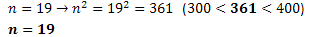# PSAT/SAT Math Attack – Raised to the (1/2) PowerToday we will be looking at an SAT Math sample question of the grid-in variety. As the only questions on the test (aside from the essay prompt) that are not multiple choice, the grid-ins can seem intimidating, but it is important to keep your cool and remember that these questions are no more complex than any others you will see on the Math section.

If [300^(1/2) < n < 400^(1/2)] and n is an integer, what is one possible value of n?

This question is pretty straightforward as long as you keep a few things in mind:

1)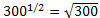Raising a number to the 1/2 power is the same thing as taking the square root, so we can think of the original inequality as: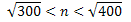We can simplify by squaring each term: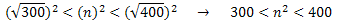2) n is an integer, which means that it is a number with fractional parts. Thus, we know our answer will not involve any decimals or fractions.

Armed with this knowledge, we can now proceed to solve the problem. The easiest method is to have your squares memorized, at least through 20. In case you do not, here are 11 through 20: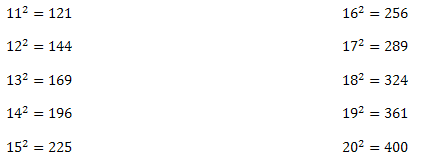Since n^2 must be between 300 and 400, we are left with only two possible solutions: n=18 or n=19. Note that many grid-in questions have more than one correct answer, so the solution can be either 18 or 19.

If you do not have these squares memorized, you will be at a disadvantage on this problem, but take heart! There is still a simple way to find the answer. You can use the math trick known as “plugging in.” All you need to do is take an educated guess at what n could be and see if your guess solves the problem. If it does, great! If not, adjust accordingly and try again.

For example, we may not know that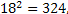but we do know that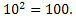This means that n > 10, so out first guess might be something like n = 15. Using a calculator (or pencil and scratch paper), we might find that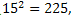which is too low. Our next guess might be 20. Multiplying out, we find that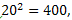which is just barely too high (remember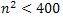). Knowing that we are closing in on our answer, our next guess will be n=19. When we plug this in, we confirm that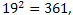which is in our range. Of course, if we had arrived at a guess n=18, that would have been correct as well. Here is a summary of what this looks like:

READ  T-Minus One: What To Do The Day Before The SAT

1) First guess: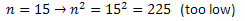2) Second guess: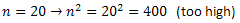3) Third guess: